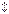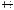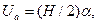# Experimental part. Order of carrying out the task 1

Order of carrying out the task 1

1. Switch on the oscilloscope. Obtain a distinct sweep trace by tuning knobs "Яркость" and "Фокус". Set the sweep trace in the centre of the screen using knobs "" and "".

2. Switch on the generator 1. Supply the signal of 400 Hz frequency from terminal "Выход 600 Ом" of the generator to inputY of the oscilloscope (on the left-side panel) through electric cable.

3. Set the switch "V/дел." in the position with maximum signal within limits of oscilloscope’s screen.

4. Locate within the screen 1-2 periods of the signal by the "ms/дел.", "µs/дел." switch.

5. Turn knob "Стаб" to the left reaching disappearance of the signal. Then turning knob "Уровень" to the right, get appearance of the signal on the screen.

6. Determine H and L in large scale graduations (fig. 6.1). Be sure that knobs "Усиление" and "Плавно" are in the right limit position (after a click).

7. Repeat measurements setting up frequency of the generator signal to be 500 Hz; 1000 Hz.

8. Calculate amplitude and effective values of the signal for all three frequencies mentioned above.Figure 6.1

The amplitude value of the signal(6.1)

where α is the coefficient of vertical deflection (position of the switch "V/дел." ). The effective value of voltage

Ueff = Ua /. (6.2)

It has to be in accordance with the reading of the generator 1 voltmeter.

9. Calculate period and frequency of the signal for all three frequencies mentioned above.

The period can be found as

T = kL , (6.3)

where k is the sweep coefficient (which corresponds to the position of the switch "ms/дел.", "µs/дел."). The frequency of the signal is calculated by the formula

ν = 1/T . (6.4)

It is needed to compare the calculated values of frequency with ones set up on the scale of the generator 1.

10. Estimate an error for one of the appointed frequencies ν1

Δν ≈ (ΔL/L1) ν1, (6.5)

where ΔL = 0.2 is the small scale graduation on the screen.

If the difference between ν1 and the calculated frequency is more than ±2Δν, attempt to find some other source of an error that could explain such a divergence.

Order of carrying out the task 2

1. Switch on the oscilloscope. Obtain a distinct sweep trace by tuning knobs "Яркость" and "Фокус". Set the sweep trace in the centre of the screen using knobs "" and "".

2. Switch on the generator 1. Supply the signal of 3 kHz frequency from terminal "Выход 600 Ом" of the generator to inputY of the oscilloscope (on the left-side panel) through electric cable.

3. Set the switch "V/дел." in the position with maximum signal within limits of oscilloscope screen.

4. Set the switch "Разверт." on the right-side panel in the lower position.

5. Switch on the generator 2. Supply the signal of 0.9 kHz frequency from terminal "Выход 600 Ом" of the generator to inputX of the oscilloscope (on the right-side panel) through electric cable.

6. Varying amplitude of the generator output signal by the knob above output terminals, get the signal not to pass the side boundaries of the screen.

7. Increase gradually frequency of the generator signal from 0.9 to 10 kHz and fix the values at which static figures (so-called Lissajous figures) are observed. Show these figures schematically in the report.

8. Make conclusion about an effect of the relationship between frequencies of generators 1 and 2 on an appearance of Lissajous figures.

Поможем в написании учебной работы
Поможем с курсовой, контрольной, дипломной, рефератом, отчетом по практике, научно-исследовательской и любой другой работой

 Дата добавления: 2015-09-04; просмотров: 242. Нарушение авторских прав; Мы поможем в написании вашей работы!
Studopedia.info - Студопедия - 2014-2022 год . (0.019 сек.) русская версия | украинская версия
Поможем в написании
> Курсовые, контрольные, дипломные и другие работы со скидкой до 25%
3 569 лучших специалисов, готовы оказать помощь 24/7